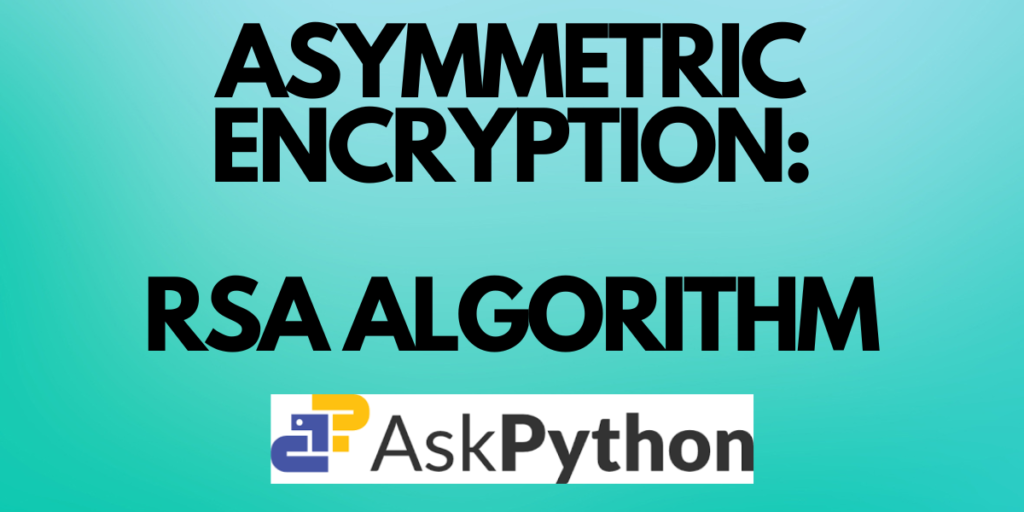# RSA Algorithm: Theory and Implementation in PythonCryptography is the practice of securing communication by using codes and ciphers. It includes a variety of techniques for converting plaintext into ciphertext, enabling secure communication, and protecting the confidentiality and integrity of data. Banking, email, e-commerce, and other industries all employ cryptography extensively. In this article you will learn about asymmetric encryption and the RSA algorithm.

Also read: A* Algorithm – Introduction to The Algorithm (With Python Implementation)

## Asymmetric Encryption

Asymmetric encryption, commonly referred to as public-key cryptography, uses two distinct keys for encryption and decryption. The public key, which is extensively used to encrypt data and is known to all, is one type of key. The private key, on the other hand, is kept private i.e., only the receiver knows it and is used to decode data.

Both, the public key and the private key should be available at both the sender’s end and the receiver’s end for the asymmetric encryption to succeed.

The encryption algorithm receives the sender’s plain text message, encrypts it using the recipient’s public key, and generates a cipher. The recipient then receives this cipher via a transmission or communication channel. The decryption process on the receiver’s end uses the decryption algorithm and the receiver’s private key to recover the original plain text message.

Asymmetric encryption typically consists of three main components:

1. Key Generation: In this step, a user generates a public-private key pair. The public key is made freely available to anyone who wants to send a message to the user, while the private key is kept secret by the user.
2. Encryption: In this step, the sender uses the recipient’s public key to encrypt the message. This ensures that only the recipient, who has the corresponding private key, can decrypt and read the message.
3. Decryption: In this step, the recipient uses their private key to decrypt the message, which was encrypted using their public key. This ensures that only the recipient can read the original message.

Although there are mathematical connections between the public key and the private key, doing so computationally is not practical. This means that anyone can encrypt data using the public key, but only the owner of the private key can decode the data.

Note: A message that is encrypted using a public key can only be decrypted using a private key, while also, a message encrypted using private key can be decrypted using a public key.

Now let us learn about the RSA Algorithm.

## RSA Algorithm

The RSA algorithm is a widely used public-key encryption algorithm named after its inventors Ron Rivest, Adi Shamir, and Leonard Adleman. It is based on the mathematical concepts of prime factorization and modular arithmetic.

The algorithm for RSA is as follows:

1. Select 2 prime numbers, preferably large, p and q.
2. Calculate n = p*q.
3. Calculate phi(n) = (p-1)*(q-1)
4. Choose a value of e such that 1<e<phi(n) and gcd(phi(n), e) = 1.
5. Calculate d such that d = (e^-1) mod phi(n).

Here the public key is {e, n} and private key is {d, n}. If M is the plain text then the cipher text C = (M^e) mod n. This is how data is encrypted in RSA algorithm. Similarly, for decryption, the plain text M = (C^d) mod n.

Example: Let p=3 and q=11 (both are prime numbers).

• Now, n = p*q = 3*11 = 33
• phi(n) = (p-1)*(q-1) = (3-1)*(11-1) = 2*10 = 20
• Value of e can be 7 since 1<7<20 and gcd(20, 7) = 1.
• Calculating d = 7^-1 mod 20 = 3.
• Therefore, public key = {7, 33} and private key = {3, 33}.

Suppose our message is M=31. You can encrypt and decrypt it using the RSA algorithm as follows:

Encryption: C = (M^e) mod n = 31^7 mod 33 = 4

Decryption: M = (C^d) mod n = 4^3 mod 33 = 31

Since we got the original message that is plain text back after decryption, we can say that the algorithm worked correctly.

Below is the Python code for the implementation of the RSA Algorithm:

```import math

# step 1
p = 3
q = 7

# step 2
n = p*q
print("n =", n)

# step 3
phi = (p-1)*(q-1)

# step 4
e = 2
while(e<phi):
if (math.gcd(e, phi) == 1):
break
else:
e += 1

print("e =", e)
# step 5
k = 2
d = ((k*phi)+1)/e
print("d =", d)
print(f'Public key: {e, n}')
print(f'Private key: {d, n}')

# plain text
msg = 11
print(f'Original message:{msg}')

# encryption
C = pow(msg, e)
C = math.fmod(C, n)
print(f'Encrypted message: {C}')

# decryption
M = pow(C, d)
M = math.fmod(M, n)

print(f'Decrypted message: {M}')
```

Output:

```n = 21
e = 5
d = 5.0
Public key: (5, 21)
Private key: (5.0, 21)
Original message:11
Encrypted message: 2.0
Decrypted message: 11.0
```

Being able to do both encryption and digital signatures is one of the RSA algorithm’s key benefits. To confirm that the message has not been tampered with, digital signatures are made by encrypting a message hash with the sender’s private key. This encryption may then be validated by anybody with access to the sender’s public key.

## Conclusion

You gained knowledge of symmetric encryption and the RSA algorithm in this article. You also saw how the RSA algorithm was implemented in Python.